Courses

# Chemistry Test 2 - Equilibrium I And II, Thermodynamics, Hydrogen, Hydrocarbons, Redox, States Of Matter, P-Block

## 30 Questions MCQ Test JEE Main Mock Test Series 2020 & Previous Year Papers | Chemistry Test 2 - Equilibrium I And II, Thermodynamics, Hydrogen, Hydrocarbons, Redox, States Of Matter, P-Block

Description
This mock test of Chemistry Test 2 - Equilibrium I And II, Thermodynamics, Hydrogen, Hydrocarbons, Redox, States Of Matter, P-Block for JEE helps you for every JEE entrance exam. This contains 30 Multiple Choice Questions for JEE Chemistry Test 2 - Equilibrium I And II, Thermodynamics, Hydrogen, Hydrocarbons, Redox, States Of Matter, P-Block (mcq) to study with solutions a complete question bank. The solved questions answers in this Chemistry Test 2 - Equilibrium I And II, Thermodynamics, Hydrogen, Hydrocarbons, Redox, States Of Matter, P-Block quiz give you a good mix of easy questions and tough questions. JEE students definitely take this Chemistry Test 2 - Equilibrium I And II, Thermodynamics, Hydrogen, Hydrocarbons, Redox, States Of Matter, P-Block exercise for a better result in the exam. You can find other Chemistry Test 2 - Equilibrium I And II, Thermodynamics, Hydrogen, Hydrocarbons, Redox, States Of Matter, P-Block extra questions, long questions & short questions for JEE on EduRev as well by searching above.
QUESTION: 1

Solution:
QUESTION: 2

### The heat of hydrogenation of 1-hexene is 126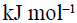. When a second double bond is introduced in the molecule, the heat of hydrogenation of the resulting compound is 230. The resulting compound (diene) is

Solution: The Diene thus formed is more stable than the initial compound (and rest of the options) due to conjugation (a conjugated diene). Hence the heat of hydrogenation is more as the product will be a thermodynamically controlled one ie a TCP.
QUESTION: 3

### Heat of hydrogenation of benzene is 51 kcal/mol and its resonance energy is 36 kcal/mol. Then the heats of hydrogenation of cyclohexadiene and cyclohexene per mole respectively are

Solution:
QUESTION: 4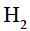is adsorbed on palladium surface.It is a case of
Solution:
QUESTION: 5

The enthalpy of fusion of water is 1.435 kcal/mol. The molar entropy change for the melting of ice at 0°C is

Solution:
QUESTION: 6
When 0.532 g of benzene (B.P.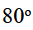C) is burnt in a constant volume system with an excess of oxygen, 22.3 KJ of heat is given out.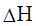for the combustion process is given by :
Solution:
QUESTION: 7
The heat released when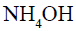& HCl neutralize is :
Solution:
QUESTION: 8
The heat of formation of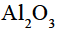is 380 kcals/mole and that of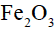is 195 kcals/mole. The heat (in kcals/mole) of the thermite reaction is
Solution:
QUESTION: 9
Enthalpy of Rhombic sulphur of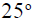C is
Solution:
QUESTION: 10
In the gaseous equilibrium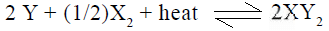the formation of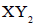will be favored by
Solution:
QUESTION: 11

The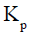for the decomposition of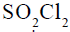(if its degree of dissociation under one atomic pressure is 90%) is

Solution: So2cl2---so2+cl2
1. 0. 0
1-.90. .90. .90
total moles at equill. = 1.90
xso2 =.90/1.90 xcl2=.90/1.90 xso2cl2= .10/1.90

kp = pso2 *pcl2 /pso2cl2
kp = .90*.90/1.90*.10 = 4.26
QUESTION: 12
The pH of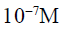HCl is
Solution:
QUESTION: 13
If the maximum concentration of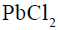in water is 0.01M at 298K, its maximum concentration in 0.1M NaCl will be
Solution:
Pbcl2 = pb +2 + 2 cl - S S 2s
Ksp = s * (2s)^2
Ksp = 0.01 * (4 * 10^-4)
Ksp = 4*10^-6
Let solubility in Nacl be s’ Pbcl2 = pb + 2cl S’ S’ 2s’ + 0.1
Neglecting 2s’ Ksp remains same 4*10^-6 = s’ * (0.1) ^2
By solving this S’ = 4 * 10^-4
QUESTION: 14

In a reversible chemical reaction at equilibrium, if the concentration of any one of the reactants is doubled, then the equilibrium constant will

Solution:
QUESTION: 15
Ammonium hydrogen sulphide is contained in a closed vessel at 313 K when total pressure at equilibrium is found to be 0.8 atm. The value of Kp for the reaction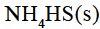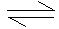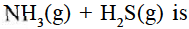Solution: If dissociation of NH4HS is x then at equilibrium,

2x=0.8 ( Using PV= nRT you can equate partial pressure to total pressure.)
so, x= 0.4 atm.
Then Kp= [P]²= (0.4)²=0.16
QUESTION: 16
Reactivity of hydrogen atoms attached to different carbon atoms in alkanes has the order
Solution:
QUESTION: 17
Reaction of ethene with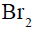in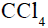gives
Solution:
QUESTION: 18
The major product obtained when isobutane is treated with chlorine in the presence of light is
Solution:
QUESTION: 19
The compound contains atoms X,Y,Z. The oxidation number of X is 2, Y is 5 and Z is –2, a possible formula of the compound is
Solution:
QUESTION: 20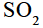acts as

Solution:
QUESTION: 21
How many allotropic forms of carbon are there ?
Solution: Diamond, Graphite, Lonsdaleite, Fullerene, Amorphous carbon, Carbon nanotubes. This is the six allotropes.
QUESTION: 22
Which of the following compounds is used as an abrasive
Solution: Silicon carbide, because used for non-ferrous metals silicon carbide can be bonded together by sintering to form very hard ceramics that are widely used in applications requiring metal clean.
QUESTION: 23

Formic acid (HCOOH) on heating with concentrated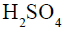gives

Solution:
QUESTION: 24
Which of the following gases is used for the purification of nickel ?
Solution:
QUESTION: 25
The most stable oxidation state of boron is
Solution:
The electronic configuration of boron is is 2 2s 2 2p 1. It has three valence electrons. Due to its small size, removal of valence electrons of boron requires very high energy. Therefore, boron does not form +3 ions, but it forms covalent compounds. Boron is less electronegative than even hydrogen. So, compounds of boron contain boron in a positive oxidation state, generally +3.
The sum of oxidation numbers of all constituent atoms of a given molecule or ion is equal to zero or the charge of the ion, respectively. Hence, the oxidation number of boron can be calculated using the formulas of its compounds as exemplified in the table.

QUESTION: 26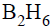reacts with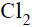to produce

Solution:
QUESTION: 27
In the Serepeck process, the bauxite ore is treated with
Solution:
QUESTION: 28
The density of methane at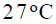and 2.0 atmoshpheric pressure is
Solution:
QUESTION: 29
A real gas obeying van der Waals equation will resemble an ideal gas if
Solution:
QUESTION: 30

CO2 can be easily liquified and even solidified because

Solution: